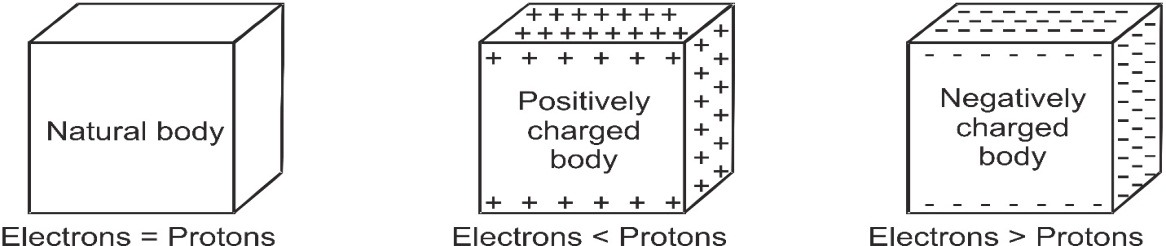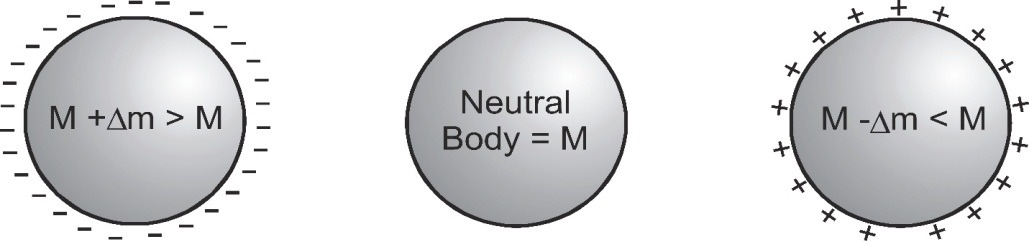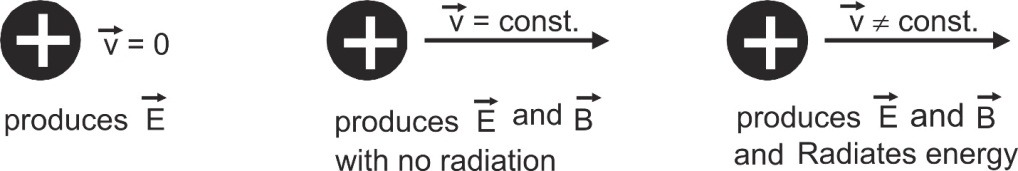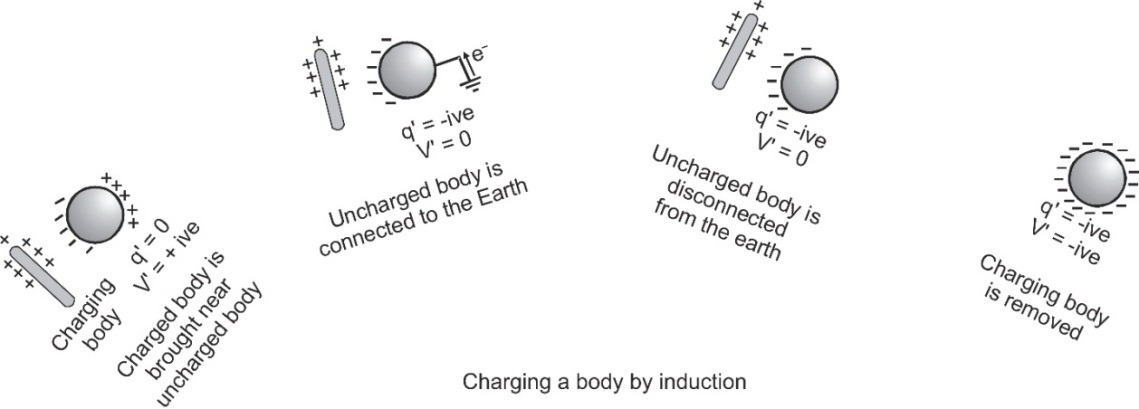# Basic Properties of Electric Charge, Definition & Solved Examples

Have you ever thought how electricity is generated? What is it made up of? Have you heard of electric charges? Let us start to know more about these bit by bit. In this article we are going to learn about Electric Charge definition and the basic properties of electric charge:

## Electric Charge Definition

Electric charge is the physical property of matter that causes it to experience a force when placed in an electromagnetic field. There are two-types of electric charges; positive and negative (commonly carried by protons and electrons respectively). [Source] The excess or deficiency of electrons in a body gives the concept of charge. A negatively charged body has excess of electrons while a positively charged body has lost some of its electrons.

[caption id="attachment_4170" align="aligncenter" width="565"]Electrons in a body defines the concept of electric charge[/caption]

## Basic Properties of Electric Charge

We have an idea about electric charge since our childhood. There are a few basic properties of electric charge listed below, some of which you might be knowing already and some you might get to know now. Let's get started:
1. Like charges repel while unlike charges attract each other. The true test of electrification is repulsion and not attraction as attraction may also take place between a charged and an uncharged body and also between two oppositely charged bodies.
2. Charge is a scalar.
3. Charge is transferable: When a charged body is put in contact with an uncharged body, the uncharged body becomes charged due to transfer of electrons from one body to the other. If the charged body is positive it will draw some electrons from the uncharged body and if it is negative then it will transfer some of its excess electrons to the uncharged body.
4. Charge is always associated with mass: Charge cannot exist without mass though mass can exist without charge. Example:
• The particles such as photon or neutrino which have zero rest mass do not have a charge.
• As charge cannot exist without mass, the presence of charge itself is a convincing proof of existence of mass. So a beam (such as β-rays, canal rays or cosmic rays) which gets deflected by electric or magnetic field will be charged and hence will consist of particles with mass. However, converse may or may not be true. If electric or magnetic field does not deflect a beam, the beam will be uncharged and so may consist of neutral particles like atoms or neutrons or electromagnetic waves like γ-rays or X-rays.
• In charging, the mass of a body changes. If electrons are removed from the body, the mass of the body will decrease and the body will become positively charged. If electrons are added to a body, the mass of the body will increase and the body will acquire a net negative charge. Due to extremely small mass of electron (= 9.1 × 10–31 kg) the change in mass of a body due to charging is negligible as compared to the mass of the body.

[caption id="attachment_4171" align="aligncenter" width="465"]Mass of a body changes according to the charge on that body - basic properties of electric charge[/caption]
5. Charge is quantized: When a physical quantity has only discrete values the quantity is said to be quantized. Millikan oil drop experiment established that the smallest charge that can exist in nature is the charge of an electron. If the charge of an electron (e = 1.6 × 10–19 C) is taken as the elementary unit, i.e., quanta of charge, the charge on a body will be an integral multiple of e. i.e., q = ± ne with n = 1, 2, 3, 4…Charge on a body can never be (2/3)e, 17.2e or 10–5 e, etc. The elementary particles such as proton or neutron are made of quarks having charge (± 1/3)e and (± 2/3)e. The quarks do not exist in free state so the quantum of charge is still e.
6. Charge is conserved: In an isolated system the total charge does not change with time, though individual charge may change. Charge can neither be created nor destroyed. Equal quantities of positive and negative charges can appear or disappear simultaneously. Conservation of charge holds good in all types of reactions i.e. chemical, nuclear or decay. No exceptions to the rule have been found.
7. Charge is invariant: This means that charge is independent of frame of reference, i.e., charge on a body does not change with speed. The charge density or mass of a body depends on speed and increases with increase in speed.
8. Accelerated charges radiate energy: Electromagnetic theory shows that a charged particle at rest produces only electric field. If the charged particle is accelerated, it produces electric and magnetic fields but does not radiate energy. In accelerated motion charge produce electric and magnetic fields and also radiate energy in the form of electromagnetic waves.

[caption id="attachment_4173" align="aligncenter" width="476"]Accelerated charges radiate energy[/caption]
9. A body can be charged by:
• Friction
• Induction
• Conduction

### Let's see these 3 in detail below:

#### Friction

In friction when two bodies are rubbed together, electrons are transferred from one body to the other. This makes one body become positively charged while the other becomes negatively charged, e.g., when a glass rod is rubbed with silk, the rod becomes positively charged while the silk is negatively charged. Clouds are also charged by friction. Charging by friction is in accordance with conservation of charge. The positive and negative charges appear simultaneously in equal amounts due to transfer of electrons from one body to the other.

#### Induction

If a charged body is brought near a neutral body, the charged body will attract opposite charge and repel similar charge present in the neutral body. This makes one side of the neutral body becomes positively charged while the other side negative.

[caption id="attachment_4174" align="aligncenter" width="580"]Charging by Induction[/caption]   Important Points
1. Inducing body neither gains nor loses charge.
2. The nature of induced charge is always opposite to that of inducing charge.
3. Induced charge can be lesser or equal to inducing charge (but never greater) and its maximum value is $q' = - q\,\,\left[ {1 - {1 \over K}} \right]$ where q is the inducing charge and K is the dielectric constant of the material of the uncharged body.
4. As K = ∞, so q' = –q for metals, i.e., in metals induced charge is equal and opposite of inducing charge.
5. Induction takes place only in bodies (either conducting or non-conducting) and not in particles.

#### Conduction

Conduction takes place when an insulated conductor is brought in contact with a charged body and it gets the same charge as the charged body. Conduction is only possible in conductors and not in insulators.

## Unit of Electric Charge

• S.I unit of electric charge is Coulomb. 1 Coulomb = 1 ampere × 1 second
• C.G.S. unit of electric charge is Static Coulomb or Franklin 1 Coulomb = 3 × 109 static Coulomb 1 Coulomb = 3 × 109 electrostatic unit (esu) of charge = ${1 \over {10}}$ electromagnetic unit of charge [esu = electrostatic unit] [emu = electromagnetic unit]
• Practical units of charge are amp × hr (= 3600 Coulomb) and Faraday (= 96500 Coulomb)

## Solved Examples

Q. Take two metallic spheres which are same in all respects. Give one of them a positive charge of Q coulomb and give the other same but negative charge. Which sphere will have a higher mass?
Ans. Negatively charged sphere will have a higher mass. This is due to increase in number of electrons to make it negatively charged.
Q. Which of the following charge is not possible?  1.6 × 10–18 C  1.6 × 10–19 C  1.6 × 10–20 C  None of these
Ans. As only 1.6 × 10–20 C is 1/10th of electric charge and hence not an integral multiple.
Q. How many electrons are present in 1 coulomb charge?
Ans.q = ne q = 1C e = 1.6 × 10–19 C So, n = q/e = 6.25 × 1018 electrons.
Q.Identify X in the following nuclear reactions- [a] 1H + 9Be → X + n [b] 12C + 1H → X [c] 15N + 1H → 4He + X
Ans. Using conservation of charge a) Charge on nucleus of X must be (because Q (n) = 0) = Q (H) + Q (Be) = 1e + 4e = 5e Thus, X is boron (B) b) Q (X) = Q (C) + Q (H) = 6e + 1e = 7e Thus, X is nitrogen (N) c) Q (X) + Q (He) = Q (N) + Q (H) Q(X) + 2e = 7e + 1e Q(X) = 6e Thus, X is carbon (C)

Rajia Bidika
Dec. 9, 2021, 7:03 p.m.
I am poor in physical science
Momo maku
Oct. 23, 2021, 8:18 a.m.
Oii sakthu
dfv
June 3, 2021, 1:59 p.m.
rtghyhyui
Rinky
May 29, 2021, 9:27 a.m.
thanks for different properties of charges
Rinky
May 29, 2021, 9:24 a.m.
nice one
May 3, 2021, 5:48 p.m.
kiliya
BTS PRINCE
March 24, 2021, 7:22 p.m.
Thanks
Kundankumar
March 15, 2021, 4:32 p.m.
9069706884
Firdous
Feb. 2, 2021, 12:47 a.m.
So nice i fully prepared
Naina
Dec. 9, 2020, 8:25 p.m.
Thanku
Bulu Acharya
June 7, 2020, 8:20 p.m.
Thanks
Jaa
Feb. 20, 2020, 10:22 p.m.
Great performance
Free Study Material